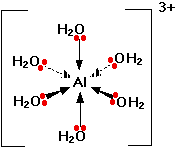# Question #f7751

Aug 27, 2017

Here's my explanation.

#### Explanation:

The formula for potassium aluminium sulfate is $\text{KAl"("SO"_4)_2·12"H"_2"O}$.

It dissociates completely in water according to the equation

$\text{KAl"("SO"_4)_2·12"H"_2"O(s)"→ "K"^"+""(aq)" + "Al"^"3+""(aq)" + "2SO"_4^"2-""(aq)" + 12"H"_2"O(l)}$

We know that $\text{K"^"+}$ and $\text{SO"_4^"2-}$ are neutral, so it must be the $\text{Al"^"3+}$ ion that is acidic.

Like many metal cations, $\text{Al"^"3+}$ is hydrated in solution.Six water molecules are strongly attached to the aluminium ion by coordinate covalent bonds.

The high positive charge on the aluminium cation pulls electron density toward itself and weakens the adjacent bonds.

Thus, it is easy for a water molecule to remove an $\text{H"^"+}$ from the hydrated ion and form $\text{H"_3"O"^"+}$.(From saylordotorg.github.io)

The solution becomes acidic.

The $\text{p} {K}_{\textrm{a}}$ of $\text{Al"^"3+}$ is 4.85, so $\text{Al"^"3+}$ has about the same acid strength as acetic acid.

The $\text{pH}$ of a 0.100 mol/L solution of $\text{Al"^"3+}$ is 2.93.